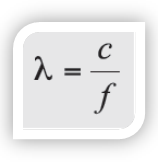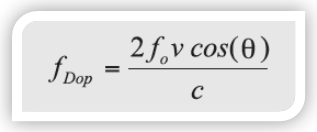My PegasusCART (0 ITEMS)

# CREDENTIALING EXAMS: How much math will you need to know?

Many people in the field of ultrasound will state there is very little mathematics on the board exams. I do not agree. I think this disparity in opinion stems from the very narrow definition of mathematics as numerical calculations. Admittedly, there are very few numerical calculations on most credentialing exams, and certainly not intensive computations that would require a calculator. However, depending on the specific credentialing test and the test version, there are approximately twenty-five to forty percent of the questions that involve some form of mathematics.The mathematics included on the credentialing exams generally does not include performing many calculations, but rather asks you to draw logical conclusions from the mathematical relationships between variables.

In other words, instead of asking you to calculate the resistance to flow for a fluid flowing through a vessel, you may be asked:

How will the resistance to flow change with ‘XYZ’ changes in the parameters that define the vessel?

Instead of asking you to calculate a Doppler shift for a given transducer frequency, given a specific angle to flow and a blood flow velocity, you may be asked:

How will the Doppler shift change if a different transducer operating frequency were used, given the same angle to flow and blood flow velocity?Answering these types of “relative” questions involves math skills, which many students have not used for a long period of time, or worse, never developed. This last point is precisely why it is so critical for you to learn (or refresh) basic mathematics.

Ultimately, I want to emphasize that credentialing and board exams are all about percentages. If you take 10% longer to complete the exam because relational math concepts are not second nature to you, or you miss 10% of the questions because of simple math comprehension errors, that 10% may be the critical difference between passing and failing. In fact, we have heard from students that most who do not pass, fail by one, two, or three questions.

Frank Miele, MSEE  President, Pegasus Lectures, Inc.graduated cum laude from Dartmouth College with a triple major in physics, mathematics, and engineering. While at Dartmouth, he was a Proctor Scholar and received citations for academic excellence in comparative literature, atomic physics and quantum mechanics, and real analysis.

This entry was posted in Exam Strategies, News and Commentary and tagged , , , . Bookmark the permalink.

### 3 Responses to CREDENTIALING EXAMS: How much math will you need to know?

1.George Allen Hides says:

Wholeheartedly agree, Frank. Understanding relationships governed by equations is power. If students default to putting on blinders when dealing with “formula-phobia”, then there is a simple math concept looming in their futures: PASS minimum minus (>=1) = FAIL. Truly great stuff in your posts, Frank. Thank you.

2.Bill says:

Thank you Frank! I appreciate your blog and recommend it to others. Not just students but old folks like me.

3.Lucas says:

Math is a tool that helps you understand what is haepnping. If all you have is a hammer than all you can do is treat everything as if it’s a nail. When you’re talking about rates, trends and curves, differential calculus is useful. When you’re trying to account for possible outcomes, probabilities and integral calculus is useful. However, as so many people who jump into trading have a poor knowledge of math, they have consolidated some of the math into equations, for example the Black Scholes equation is the result of integral calculus on a logarithmic gaussian normal distribution of possible future values for the underlying stock but many people don’t know that and just use the pre-programmed function on their business calculator. Similarly, the DDM, Gordon’s equation, ordinary annuity equation, NPV, IRR, bond yield, bond market price, etc. are all just the application of the summation of a geometric sequence that you learned in junior high and the future value equation that you also learned in junior high but so few people actually remember it that they simplified it into equations to memorize. The more you understand about math, the more these concepts will make sense but you can fake it as many people do, with just memorizing equations and learning how to use tables and charts that are derived from the math, the only loss is your understanding of what is haepnping.You might want to start by spelling percentage correctly and realizing that percentage is just part of algebra.